Chapter 12. Acids and Bases

# The pH Scale

Learning Objectives

1. Define pH.
2. Determine the pH of acidic and basic solutions.

As we have seen, [H+] and [OH] values can be markedly different from one aqueous solution to another. So chemists defined a new scale that succinctly indicates the concentrations of either of these two ions.

pH is a logarithmic function of [H+]: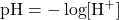pH is usually (but not always) between 0 and 14. Knowing the dependence of pH on [H+], we can summarize as follows:

• If pH < 7, then the solution is acidic.
• If pH = 7, then the solution is neutral.
• If pH > 7, then the solution is basic.

This is known as the pH scale. You can use pH to make a quick determination whether a given aqueous solution is acidic, basic, or neutral.

Example 12.13

Label each solution as acidic, basic, or neutral based only on the stated pH.

1. milk of magnesia, pH = 10.5
2. pure water, pH = 7
3. wine, pH = 3.0

Solution

1. With a pH greater than 7, milk of magnesia is basic. (Milk of magnesia is largely Mg(OH)2.)
2. Pure water, with a pH of 7, is neutral.
3. With a pH of less than 7, wine is acidic.

Test Yourself
Identify each substance as acidic, basic, or neutral based only on the stated pH.

1. human blood, pH = 7.4
2. household ammonia, pH = 11.0
3. cherries, pH = 3.6

1. basic
2. basic
3. acidic

Table 12.3 “Typical pH Values of Various Substances” gives the typical pH values of some common substances. Note that several food items are on the list, and most of them are acidic.

Table 12.3 Typical pH Values of Various Substances
Substance pH
stomach acid 1.7
lemon juice 2.2
vinegar 2.9
soda 3.0
wine 3.5
coffee, black 5.0
milk 6.9
pure water 7.0
blood 7.4
seawater 8.5
milk of magnesia 10.5
ammonia solution 12.5
1.0 M NaOH 14.0

pH is a logarithmic scale. A solution that has a pH of 1.0 has 10 times the [H+] as a solution with a pH of 2.0, which in turn has 10 times the [H+] as a solution with a pH of 3.0 and so forth.

Using the definition of pH, it is also possible to calculate [H+] (and [OH]) from pH and vice versa. The general formula for determining [H+] from pH is as follows: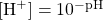You need to determine how to evaluate the above expression on your calculator. Ask your instructor if you have any questions.

The other issue that concerns us here is significant figures. Because the number(s) before the decimal point in a logarithm relate to the power on 10, the number of digits after the decimal point is what determines the number of significant figures in the final answer:Example 12.14

What are [H+] and [OH] for an aqueous solution whose pH is 4.88?

Solution
We need to evaluate the following expression: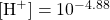Depending on the calculator you use, the method for solving this problem will vary. In some cases, the “−4.88” is entered and a “10x” key is pressed; for other calculators, the sequence of keystrokes is reversed. In any case, the correct numerical answer is as follows: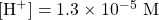Because 4.88 has two digits after the decimal point, [H+] is limited to two significant figures. From this, [OH] can be determined: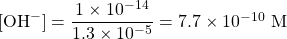Test Yourself
What are [H+] and [OH] for an aqueous solution whose pH is 10.36?

[H+] = 4.4 × 10−11 M; [OH] = 2.3 × 10−4 M

There is an easier way to relate [H+] and [OH]. We can also define pOH similar to pH: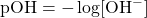(In fact, p“anything” is defined as the negative logarithm of that anything.) This also implies that: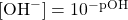A simple and useful relationship is that, for any aqueous solution: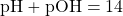This relationship makes it simple to determine pH from pOH or pOH from pH and then calculate the resulting ion concentration.

Example 12.15

The pH of a solution is 8.22. What are pOH, [H+], and [OH]?

Solution
Because the sum of pH and pOH equals 14, we have: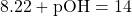Subtracting 8.22 from 14, we get: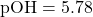Now we evaluate the following two expressions: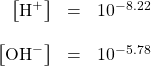So: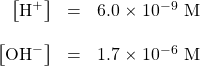Test Yourself
The pOH of a solution is 12.04. What are pH, [H+], and [OH]?

pH = 1.96; [H+] = 1.1 × 10−2 M; [OH] = 9.1 × 10−13 M

Key Takeaways

• pH is a logarithmic function of [H+].
• [H+] can be calculated directly from pH.
• pOH is related to pH and can be easily calculated from pH.

Exercises

# Questions

1. Define pH. How is it related to pOH?
2. Define pOH. How is it related to pH?
3. What is the pH range for an acidic solution?
4. What is the pH range for a basic solution?
5. What is [H+] for a neutral solution?
6. What is [OH] for a neutral solution? Compare your answer to Exercise 5. Does this make sense?
7. Which substances in Table 12.3 “Typical pH Values of Various Substances” are acidic?
8. Which substances in Table 12.3 are basic?
9. What is the pH of a solution when [H+] is 3.44 × 10−4 M?
10. What is the pH of a solution when [H+] is 9.04 × 10−13 M?
11. What is the pH of a solution when [OH] is 6.22 × 10−7 M?
12. What is the pH of a solution when [OH] is 0.0222 M?
13. What is the pOH of a solution when [H+] is 3.44 × 10−4 M?
14. What is the pOH of a solution when [H+] is 9.04 × 10−13 M?
15. What is the pOH of a solution when [OH] is 6.22 × 10−7 M?
16. What is the pOH of a solution when [OH] is 0.0222 M?
17. If a solution has a pH of 0.77, what is its pOH, [H+], and [OH]?
18. If a solution has a pOH of 13.09, what is its pH, [H+], and [OH]?

1. pH is the negative logarithm of [H+] and is equal to 14 − pOH.
1. pH < 7
1. 1.0 × 10−7 M
1. Every entry above pure water is acidic.
1. pH of 3.46
1. pH of 7.79
1. pOH of 10.54
1. pOH of 6.21
1. pOH = 13.23; [H+] = 1.70 × 10−1 M; [OH] = 5.89 × 10−14 M

1. Actual values may vary depending on conditions.